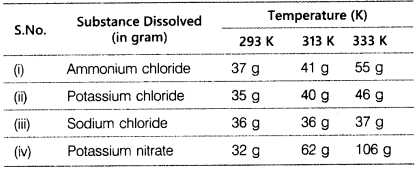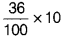# What is the effect of temperature on the solubility of a salt?

Rama tested the solubility of four substances at different temperatures and recorded the following observations regarding few gram of each substance dissolved in 100 g of water to form a saturated solution.1. Which soution is least soluble at 293 K?
2. Which substance shows maximum change in its solubility when the temperature is raised from 293 K to 313 K?
3. Find the amount of ammonium chloride that will separate out when 55 g of its solution at 333 K is cooled to 293 K.
4. What is the effect of temperature on the solubility of a salt?
5. What mass of sodium chloride would be needed to make a saturated solution in 10 g of water at 293 K?
1. Potassium nitrate
2. Potassium nitrate
3. At 333 K, ammonium chloride dissolved per 100 g = 55 g
At 293 K, ammonium chloride dissolved per 100 g = 37 g
When solution is cooled from 333 K to 293 K,
amount of ammonium chloride that will separate out = 55 g - 37g = 18 g
4. Solubility of a salt (solid) increases with rise in temperature and vice-versa.
5. At 293 K, amount of NaCI dissloved in 100 g water = 36 g
∴ At 293 K, amount of NaCI dissolved in 10 g water == 3.6 g